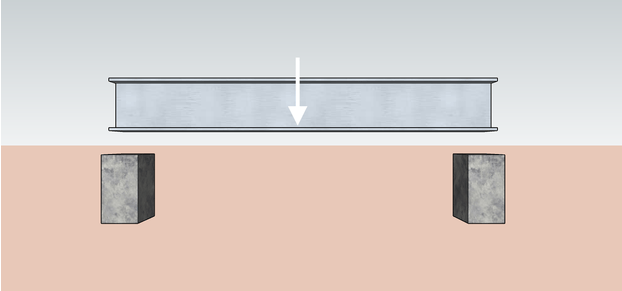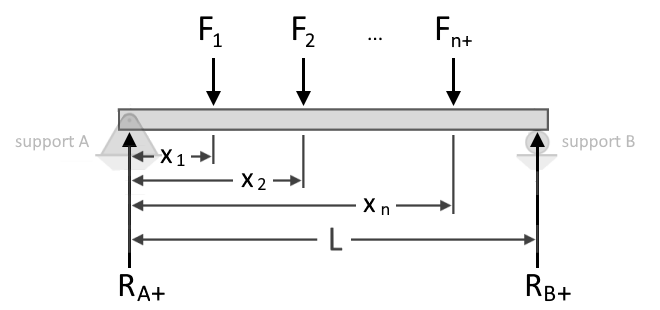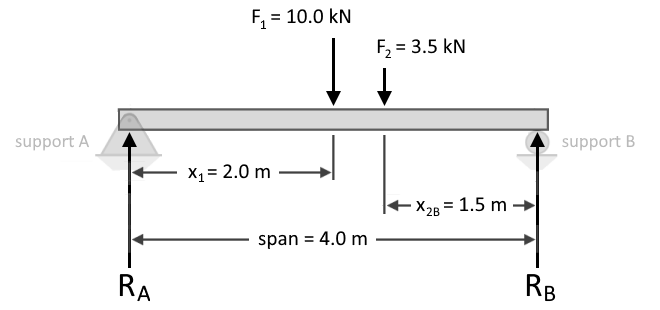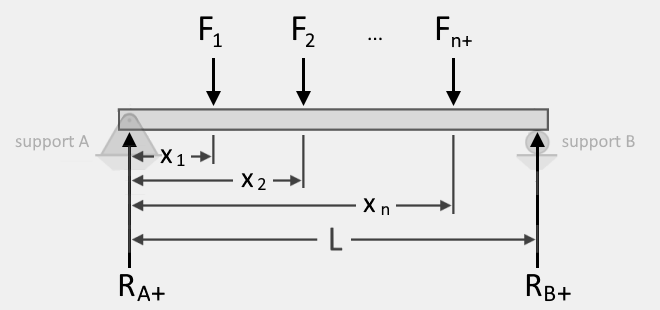# Beam Load Calculator

Created by Kenneth Alambra
Reviewed by Dominik Czernia, PhD candidate and Jack Bowater
Last updated: Apr 06, 2022

This beam load calculator will help you determine the reactions at the supports of a simply-supported beam due to vertical point loads or forces. In this calculator, you will learn what support reaction is and learn the basics of how to calculate beam load capacity.

Knowing how to find support reactions is a great place to start when analyzing beams, such as when determining beam deflection. Keep on reading to learn more.

## What is a support reaction?

According to Newton's third law of motion, every force on an object has an equal and opposite reaction. If you try to push on to something, let's say a wall, it feels like the wall is also pushing back at you. That is exactly the phenomena Newton's third law of motion describes.

In engineering, structural members such as beams and columns interact with each other at the points where they meet. Imagine a beam that is being supported in place by two columns. The beam's weight pushes down on the columns, and, because of Newton's third law of motion, we can then also say that the columns exert an equivalent opposite reacting force back on the beam. We call these reacting forces support reactions.On a simply-supported beam, the support reactions at each end of the beam can either be equal to each other or of different values. Their values depend on the beam's applied loads. If more loads are found at a closer distance to one support, that support experiences more force and therefore experiences a greater reaction.

## How to calculate support reactions in the beam?

Since support reactions act in the opposite direction as the force, we can say that the whole system is in equilibrium. That means the beam is not moving and the summation of forces and moments result in zero. By equating the moments due to load to the moments due to support reactions, we can then determine the reactions at the supports.Just like when calculating torque, we can also perform a summation of moments at each supports to solve for the reactions. Below, we express a summation, Σ, of the moments at support A to find the reaction at support B, denoted as RB, as shown below:

Σ(F * x) - (RB * span) = 0

(F1 * x1) + (F2 * x2) + (F3 * x3) + ... + (Fn * xn) - (RB * span) = 0

where:

• F, F1, F2, F3 and Fn - The point loads on the beam at distances x, x1, x2 x3 and xn from support A, respectively;
• RB - Reaction at support B; and
• span - Length of beam between support A and support B.

By rearranging the equation, we can isolate RB as follows:

RB * span = (F1 * x1) + (F2 * x2) + (F3 * x3) + ... + (Fn * xn)

RB = ((F1 * x1) + (F2 * x2) + (F3 * x3) + ... + (Fn * xn)) / span

Now that we have an expression to find RB, and since we know that the total applied forces are equal to the reactions' total, we can now also find the reaction at support A RA, using the following equations:

 Σ(F) = Rᴀ + Rʙ

RA = Σ(F) - Rʙ

## Sample calculation of support reaction

Suppose we have a 4.0-meter long simply-supported beam with an applied 10.0 kilonewtons (kN) point load 2.0 meters from support A and another applied 3.5 kN point load 1.5 meters from support B, as shown below:To calculate for RB, we formulate the equation of moment equilibrium as follows:

RB = (F1 * x1 + F2 * x2) / span

RB = (10 kN * 2.0 m + 3.5 kN * (4.0 m - 1.5 m)) / 4.0 m

RB = (20 kN-m + 3.5 kN * 2.5 m) / 4.0 m

RB = (20 kN-m + 8.75 kN-m) / 4.0 m

RB = 7.1875 kN

By performing a summation of forces, we obtain:

 Σ(Fn) = 0

 F1 + F2 + (-Rᴀ) + (-Rʙ) = 0

 10 kN + 3.5 kN + (-Rᴀ) + (-7.1875 kN) = 0

RA = 10 kN + 3.5 kN - 7.1875 kN

RA = 6.3125 kN

Please note that for this summation, we have considered all downward forces as positive and all upward forces as negative. Based on our calculations above, we have now obtained the reactions at supports A and B to be 6.3125 kN and 7.1875 kN, respectively.

Also, please take note that in this example and in the beam load calculator, we assumed that the beam is weightless. However, if the beam's weight is indicated, you can consider the beam's weight as another downward point load at the center, or centroid, of the beam.

## Using our beam load calculator

Our calculator is easy and simple to use. All you have to do is input the span of the beam, the magnitude of the point loads, and their distances from support A. At first, you will only see fields for two loads (Load 1 and Load 2), but once you enter a value for x2, the fields for Load 3 will show up, and so on.

If you want to enter an upward load, simply enter a negative value for the load magnitude. In total, you can input up to 11 point loads in our beam load calculator.

Now that you have learned how to calculate beam load capacity by determining the reactions at the supports, perhaps you might also want to learn more about .

Kenneth Alambra
Beam detail
Span, L
ftF₁
lbf
Distance from support A, x₁
ft
F₂
lbf
Distance from support A, x₂
ft
Output values
Show values in...
pounds-force (lbf)
With the beam fully loaded:

▪ Support reaction at A is Rᴀ = 3.3333 lbf
▪ Support reaction at B is Rʙ = 1.6667 lbf
People also viewed…

### Circumference

Use this free circumference calculator to find the area, circumference and diameter of a circle.

### Discount

Discount calculator uses a product's original price and discount percentage to find the final price and the amount you save.

### Limestone

Find the volume and weight of limestone rocks you will need for your project using this easy-to-use limestone calculator.

### Pipe volume

Use the pipe volume calculator to find what is the volume of a pipe and how much the liquid inside it weighs.